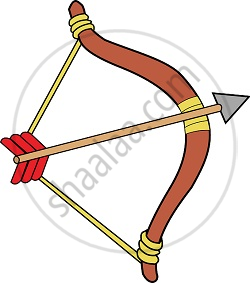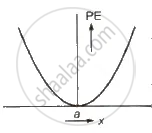# The Concept of Potential Energy

#### description

• Notion of Potential Energy

## The concept of Potential energy• Potential energy is the ‘stored energy’ by virtue of the position or configuration of a body.

• Physically, the notion of potential energy is applicable only to the class of forces where work done against the force gets ‘stored up’ as energy.

• Mathematically, the potential energy V(x) is defined, if the force F(x) can be written as

F(x)=-(dv)/(dx)
∫_(x_i)^(x_f) F(x)=V_i-V_f

This relation is valid only for Conservative Forces.
"The energy possessed by any object by virtue of its position or configuration is called its potential energy."

There are three important types of potential energies:
(i) Gravitational Potential Energy: If a body of mass m is raised through a height h against gravity, then its gravitational potential energy = mgh,(ii) Elastic Potential Energy: If a spring of spring constant k is stretched through a distance x. then elastic potential energy of the spring = 1/2 kx^2

The variation of potential energy with distance is shown in figure.

Potential energy is defined only for conservative forces. It does not exist for non-conservative forces.

Potential energy depends upon frame of reference.

(iii) Electric Potential Energy: The electric potential energy of two point charges q_1 and q_2. separated by a distance r in vacuum is given by

U = 1 / (4πΣ0) * (q_1q_2) / r

Here 1 / (4πΣ0) = 9.0 * 1010 (Nm^2)/ C^2  constant.

If you would like to contribute notes or other learning material, please submit them using the button below.